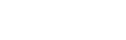# Physics Paper 3 Questions and Answers - Form 4 Term 1 Opener Exams 2023

INSTRUCTIONS TO CANDIDATES:
• You are supposed to spend the first 15 minutes of the 2 ½ hours allowed for this paper reading the whole paper carefully before commencing your work.
• Marks are given for a clear record of the observation actually made, their suitability, accuracy and the use made of them.
• Candidates are advised to record their observations as soon as they are made
• Non-programmable silent electronic calculators may be used.
1. You are provided with the following apparatus:
• A half-metre rule.
• A knife edge.
• A 50g mass.

Procedure
1. Write down the mass of the half-metre rule as labelled on it.
Mass R………………………….kg (1 mark)
2. Using a loop of thread suspend the 50g mass at the 49.5cm mark on the half-metre rule.
3. With the 50g mass fixed at that position adjust the position of the half-metre rule on the knife edge until it balances horizontally as shown in figure 1.4. At the balance position, read off the length x and y and record in table 1.
5. Move the suspended mass 2cm towards the centre of the rule and repeat parts (iii) and (iv) to obtain other values of x and y so as to complete table1. (6 marks)
 Position of the mass of 50g x (cm) y (cm) 49.5 cm mark 47.5 cm mark 45.5 cm mark 43.5 cm mark 41.5 cm mark 39.5 cm mark 37.5 cm mark 35.5 cm mark
6. Plot a graph of y (cm) against x (cm). (5 marks)
8. The relationship between y and x is given by y=  50/Px + C where P is a constant and C is the distance of the centre of gravity of the rule from the ‘zero’ end. From your graph determine;
1. The constant P. (3 marks)
2. The position of the centre of gravity of the rule. (2 marks)
2.
1.
1. You are provided with the following apparatus:
• Resistance wire fitted on a scale labelled MN
• Switch
• Voltmeter
• Ammeter
• Two dry cells
• Six connecting wires
1. Set up the apparatus as shown in the figure below;2. Remove the crocodile clip to the resistance wire such that MN and close the switch. Record the voltmeter reading.
Y=………………………………………..V (1 mark)
3. Attach the crocodile clip to the resistance wire such that L=10cm
4. Record the voltmeter and ammeter readings in the table below.
5. Repeat the procedure in (iii) and (iv) for L=20cm, 30cm, 50cm, and 80cm
6. Complete the table below; (5 marks)
 Length L=cm 10 20 30 50 80 Current I (A) P.d (V) Y-V V   Y-V R = V/I
2. Plot a graph of R (vertical axis) against  V     (5 marks)
Y-V
3. Determine the slope m of the graph (2marks)
4. The graph is given by the equation R= 5mv + d  determine the value of m and d (2 marks)
y − v
2. You are provided with the following set of apparatus
• A metre rule
• A white screen
• A candle
• Lens and lens holder

Procedure
1. Set up the apparatus as shown2. Starting with L1 = 30cm, adjust the position of the candle in order to obtain a sharp image on the screen. Record the value of L2………………………………………. (1mk)
3. Repeat the procedure for L1 = 60cm
4. Fill and complete for values of L1/L2 below. (3 marks)
 L1cm L2(cm) L1/L2 30 60
5. Given the equation
F1   L1
(m+1)
When m = magnification determine the value of focal length f, (2 marks)

CONFIDENTIAL

Question 1

Each candidate will require:

• A half – metre rule.
• A 50g mass
• A knife edge.

Question 2

Each candidate will require:

• A resistance wire/SWG 32-nichrome mounted on a millimeter scale labelled MN.
• A switch
• A voltmeter (O1A)
• An ammeter (O1A)
• Two dry cells size D (New)
• Six connecting wires at least 3 with crocodile clips
• White screen
• Candle
• Match box (to be shared)
• Convex lens (f=20cm)
• Lens holder.## MARKING SCHEME

1. i) Mass 0.057 kg ✓1    Mark according to the material of metre rule in your school
v) Table 1
 Position of the mass of 50g x (cm) y (cm) 49.5 cm mark 13.5 36.0 47.5 cm mark 12.5 35.0 45.5 cm mark 10.5 33.7 43.5 cm mark 10.2 33.2 41.5 cm mark 9.2 32.3 39.5 cm mark 8.0 31.5 37.5 cm mark 7.9 30.6 35.5 cm mark 5.7 29.8
Maximum 6 marks
NB: These values may be affected by the rule used
vi) Plotting ✓2
Axes✓1
Scale ✓1
Line✓1
vii)  36-32.3   ✓1=  3.7✓1
13.5-9.2           4.3
= 0.86047 ✓1
viii) I) y = 50/P x+C
50/P = slope  ✓
50/P = 0.86047  ✓
P =      50      = 58 ✓
0.86047
(NB: P should equal or be close to the candidates mass in (i))
II)  C = 25  ✓ ± 1
Centre of gravity (y-intercept)  ✓
2.
1.
1. ii) V=2.7volts
vi)
 Length L=cm 10 20 30 50 80 Current I (A) 0.86 0.48 0.43 0.255 0.205 P.d (V) 2.1 2.3 2.4 2.5 2.6 Y-V 0.6 0.4 0.3 0.2 0.1 V   Y-V 3.5 5.75 8 12.5 26 R = V/I 2.442 4.792 5.581 9.804 12.683
vii)
2.3. Slope M =Ω = 12.75Ω✓1(values extracted from the graph)
4. d = 2.6Ω intercept on R axis✓1
m = Gradient x 5✓1 = (12.75 x 5)Ω = 63.75Ω✓
2. (iv)
 L1cm L2(cm) L1/L2 30 50.0 ± 2 0.60 60 27.5 ± 2 2.18
f1 =   L1   =  30  =  18.75
(m+1)    1.6
f2 =  60    = 18.75
3.18
f1 + f2 = 18.75+18.87 = 18.81
2                   2

• ✔ To read offline at any time.
• ✔ To Print at your convenience
• ✔ Share Easily with Friends / Students